# The Table Toolbar

This toolbar provides single-click access to the Plot Menu commands. These are used to plot data from tables.

Figure 4-5. The QtiPlot Table ToolbarTable 4-21. Table toolbar commands.

IconCommandKeyDescription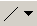Line Plots Line, Horizontal Steps, Vertical Steps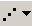Scatter Plots Scatter, Vertical Drop Lines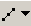Line & Symbol Plots Line+Symbol, Spline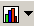Bar Chart Plots Columns, Rows, Stack Column, Stack Bar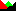plot -> Area Plots selected data using area style.plot -> 2D Color Pie Chart Plots selected data using pie style.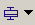Statistical Plots Box, Interval, Violin, Histogram, Histogram + Probabilities, Stacked Histogram, Pareto Chart - Binned Data, Pareto Chart - Raw Data, Bland-Altman plot, Q-Q plot, Stem-LeafVector Plots Vectors XYXY. Vectors XYAM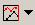Special Line/Symbol Plots Double-Y, Waterfall, Zoom, Vert. 2 Layer, Horiz. 2-Layer, 4 Layer, Stacked Layer, Custom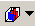3D Plots Bars, Ribbons, Scatter, Trajectory

Table 4-22. Line Plots

IconCommandKeyDescription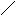plot -> Line Plots selected data using line style.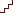plot -> Horizontal Steps Plots selected data using horizontal steps style.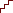plot -> Vertical Steps Plots selected data using vertical steps style.

Table 4-23. Scatter Plots

IconCommandKeyDescriptionplot -> Scatter Plots selected data using scatter style.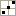plot -> Scatter Central Plots a graph of the selected data columns using "Scatter" style and the X and Y axes located in the middle of the layer.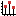plot -> Vertical Drop Lines Plots selected data using vertical drop lines style.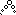plot -> Bubble Plots selected data using indexed sizes for the plot symbols.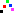plot -> Color Mapped Plots selected data using a default color map for the plot symbols.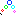plot -> Bubble + Color Mapped Plots selected data using indexed sizes and a default color map for the plot symbols.

Table 4-24. Line & Symbol Plots

IconCommandKeyDescription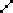plot -> Line+Symbol Plots selected data using line+symbol style.plot -> Spline Plots the selected data columns using spline style.

Table 4-25. Bar Chart Plots

IconCommandKeyDescription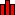plot -> Columns Plots selected data using columns style.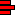plot -> Rows Plots selected data using rows style.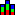plot -> Special Bar/Column -> Stack Column Plots selected data using stack column style.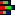plot -> Special Bar/Column -> Stack Bar Plots selected data using stack row style.

Table 4-26. Statistical Plots

IconCommandKeyDescriptionplot -> Box Plot Plots selected data using Box style.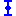plot -> Interval Plot Plots selected data using the interval style.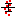plot -> Scatter Interval Plots selected data using the scatter interval style.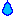plot -> Violin Plot Plots selected data using the violin style.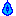plot -> Violin with Box Plots selected data using the violin with box style.plot -> Histogram Plots selected data using histogram style.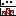plot -> Histogram + Probabilities Plots selected data using histogram style. Additionally, it plots a cumulative sum of observations in a second graph layer.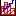plot -> Histogram + Probabilities (Double-Y) Plots selected data using histogram style. Additionally, it plots a cumulative sum of observations using a vertical steps style.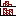plot -> Stacked Histogram Plots selected data using stacked histogram style.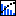plot -> Pareto Chart - Binned Data Plots a Pareto chart of the selected binned data column. The input data typically consists of a text column containing the categories/items and one of numbers containing the corresponding counts or bins. You must select one or two columns.plot -> Pareto Chart - Raw Data Plots a Pareto chart of the selected column containing raw data, typically text: categories, items, etc...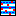plot -> Bland-Altman Plot... Opens the Bland-Altman Plot dialog that allows to create and customize a Bland-Altman plot of the selected data columns in the active table window.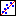plot -> Q-Q Plot... Opens the Q-Q Plot dialog that allows to create and customize a Q-Q plot of the selected data column in the active table window.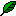plot -> Stem and Leaf Plots selected data using Stem and Leaf style.

Table 4-27. Vector Plots

IconCommandKeyDescription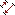plot -> Vectors XYXY Plots selected data using xyxy vector style.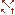plot -> Vectors XYAM Plots selected data using xyam vector style.

Table 4-28. Special Line/Symbol Plots

IconCommandKeyDescriptionPlot -> Multi-Curve -> Double-Y Plots selected data using two different Y axes.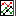Plot -> Multi-Curve -> 3Y: Y-YY Plots selected data using three different Y axes.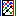Plot -> Multi-Curve -> 4Y: YY-YY Plots selected data using four different Y axes.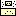Plot -> Multi-Curve -> Zoom Plots selected data in one plot layer along with a magnified region of that data in another plot layer on the same graph window.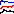Plot -> Multi-Curve -> Stack Lines by Y Offsets Creates a new graph window containing a Stacked Lines plot generated from data in the selected columns. Each column is drawn as a separate curve which has a vertical offset from the previous one.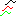Plot -> Multi-Curve -> Waterfall Creates a Waterfall plot from a series of selected columns.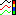Plot -> Multi-Curve -> Colormapped Lines Creates a new graph window displaying each selected data set as a line curve and a color scale legend that maps the colors of the curves to the column indices.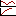plot -> Vertical 2 Layers Plot 2 vertically arranged layers on the same graph window.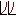plot -> Horizontal 2 Layers Plot 2 horizontally arranged layers on the same graph window.plot -> 4 Layers Plot 4 layers on the same graph window.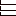plot -> Stacked Layers Plot a stacked layer for each selected column, all on the same graph window.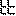plot -> Custom Layout... Opens the Arrange Layers dialog to set up custom layouts.

Table 4-29. 3D Plots

IconCommandKeyDescription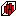plot -> Bars Plots selected data using 3D bars style.plot -> Ribbons Plots selected data using 3D ribbons style.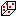plot -> Scatter Plots selected data using 3D scatter style.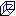plot -> Trajectory Plots selected data using trajectory style.